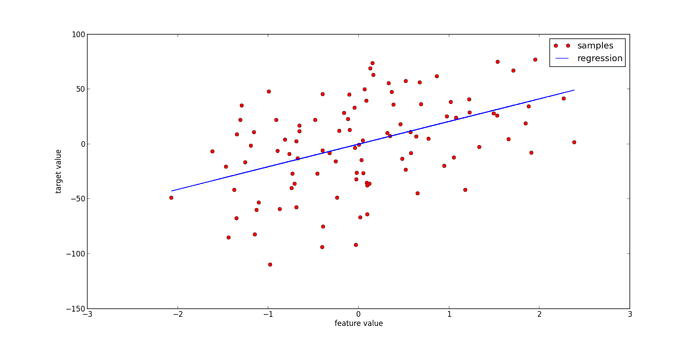# Linear Regression with Measurement Errors

Hello,

I have a set of data, two columns, and each datum has its measurement error like illustration shows below:
x | y
--------------|-----------------
x1+/-xe1 | y1+/-ye1
. | .
. | .
-------------------------------
Now, I intend to find a best fitting line with those data.
I just ask where I can find the sources or exact formulae that I can
calculate the slope and the intercept even the slope variance and intercept variance.

Thank you so much,
Han

mfb
Mentor
Every statistics textbook should have the formulas, every statistics tool will have them, for a simple least square approximation you can also follow the links at the Wikipedia article.

Every statistics textbook should have the formulas, every statistics tool will have them, for a simple least square approximation you can also follow the links at the Wikipedia article.
Thank you !

FactChecker
Gold Member
mfb
Mentor
I don't know what you call "standard", as long as the uncertainties can be treated as uncorrelated Gaussians this looks pretty regular to me.

FactChecker
Gold Member
I don't know what you call "standard", as long as the uncertainties can be treated as uncorrelated Gaussians this looks pretty regular to me.
The sum-square function being minimized is different when X measurements have errors. The error in the X measurement should not be treated as an error of the Y variable. The errors should not be added since there is a significant difference between 2 independent random error variables being 1 σ from 0 versus 1 variable being 2 σ from 0.
From the second figure of wikipedia link above: "The bivariate (Deming regression) case of total least squares. The red lines show the error in both x and y. This is different from the traditional least squares method which measures error parallel to the y axis. The case shown, with deviations measured perpendicularly, arises when x and y have equal variances."

The Deming regression reminds me of the Principle Component Analysis. I can't immediately see the difference.
Apparently the Demming Regression can be done in the language R after installing the mcr package. See https://www.r-bloggers.com/deming-and-passing-bablok-regression-in-r/. I have no experience with it.

PS. It may be advisable to rescale the X and/or Y variables so that their sample variances are equal. If these algorithms minimize a total sum-squared errors, it may be necessary that the variance of both variables be equal. However, the algorithms might already take care of this.

Last edited:
Svein
Don't forget to compute the correlation coefficient (r2) when you are through. The linear regression formula gives you an answer every time - even if it is clearly wrong. I have seen researchers in the softer disciplines using linear regression on every set of data they have in the hope of finding some significance and getting excited over an r2 of 0.01(!).mfb
Mentor
Guess the correlation

With given uncertainties, chi^2/ndf shows how reasonable the linear fit is.
The sum-square function being minimized is different when X measurements have errors. The error in the X measurement should not be treated as an error of the Y variable. The errors should not be added since there is a significant difference between 2 independent random error variables being 1 σ from 0 versus 1 variable being 2 σ from 0.
No one suggested that. You have to find the point on the line that fits best given the two independent uncertainties (in x and y). That is a single analytic expression.

FactChecker